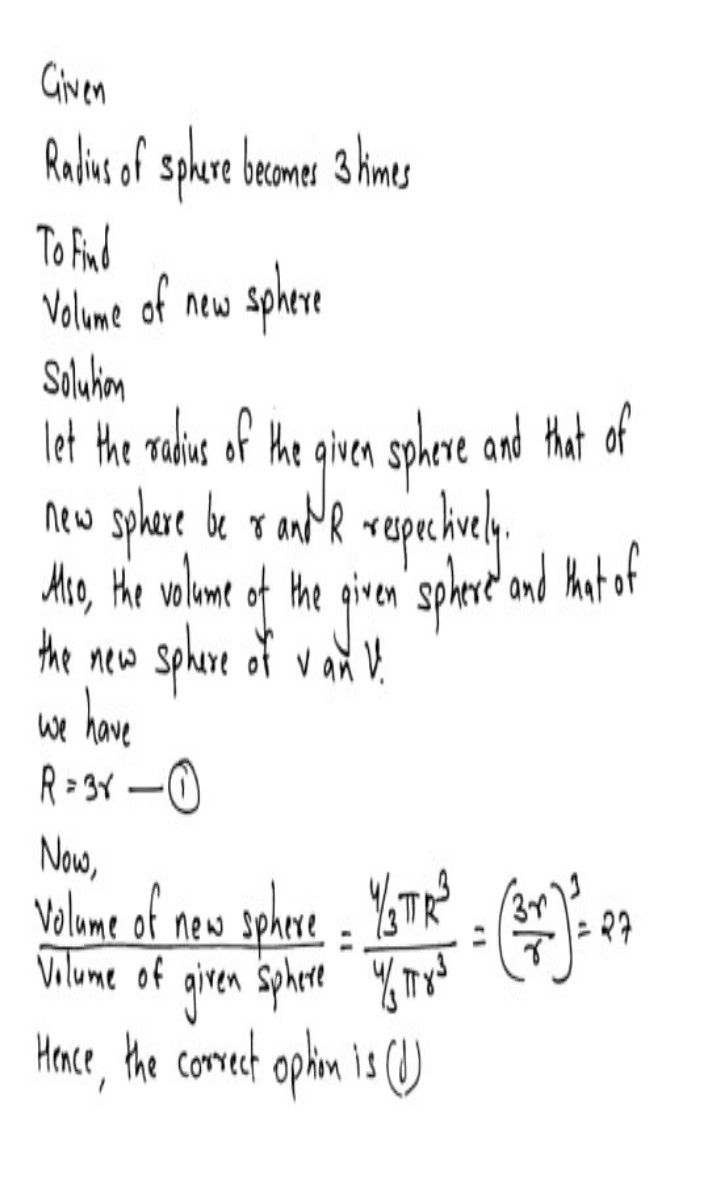Symbol
ProblemIf the radius of a sphere becomes $3$ times, then its volume will $become:$ $\left(a\right)$ $3$ times $\left(b\right)$ $6$ times (c) $9$ times $\left(\right)$ $27$ times.
Calculus
SolutionQanda teacher - RAJKUMAR